# Test: Flow Over Notches & Weirs

## 7 Questions MCQ Test Topicwise Question Bank for Civil Engineering | Test: Flow Over Notches & Weirs

Description
Attempt Test: Flow Over Notches & Weirs | 7 questions in 20 minutes | Mock test for Civil Engineering (CE) preparation | Free important questions MCQ to study Topicwise Question Bank for Civil Engineering for Civil Engineering (CE) Exam | Download free PDF with solutions
QUESTION: 1

### Consider the following measuring structures: 1. Submerged broad-crested weir 2. Free broad-crested weir 3. Free sharp-crested weir 4. Free ogee spillway The correct sequence of the increasing order of the discharge coefficient of these structures is

Solution:

For ogee spillway, Cd1 = 1.19 Cds
Cds is the discharge coefficient for sharp - crested weir. So 4 will come after 3.
The classification of weir is: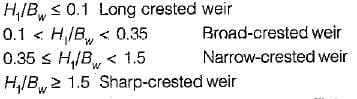Further submerged weirs have lesser coefficient of discharge compared to free weirs. So, 2 will come after 1.

QUESTION: 2

### Match List-I with List-ll and,select the correct answer using the codes given below the lists: List-I A. Rehbock formula B. Francis formula C. A special trapezoidal weir D. Linear proportional weir List-lI  1. Sutroweir 2. Rectangular suppressed weir 3. Rectangular side-contracted weir 4. Cippolietti weir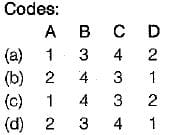Solution:

(i) Rehbock’s formula is an empirical formula for calculating discharge in rectangular suppressed weir. It is given by,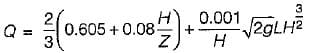Where L is the length of weir, H is head in meters and Z is the crest height in meters,
(ii) Francis’ formula is one of the commonly used empirical formula for computing the discharge over sharp or narrow crested weirs with or without end contraction. Francis proposed the following formulae for the discharge over rectangular weir.
(a) When the velocity of approach is not considered,
Q = 1.84(L - 0.1 nH)H3/2

(b) With the velocity of approach is taken into account,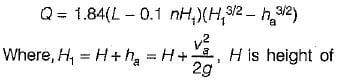water surface above the crest of weir, and n is the number of end contractions.

QUESTION: 3

### The head over a V notch at the end of a channel is 75 cm. If an error of 0.15 cm is possible in the measurement of head, the percentage error. in computing the discharge will be

Solution:

Percentage error discharge over a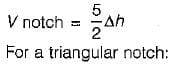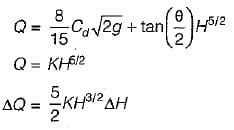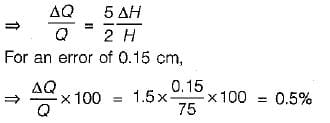Similarly percentage error in discharge in a rectangular weir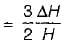QUESTION: 4

A hemispherical tank of radius R has an orifice of cross - sectional area ‘a’ at its bottom and is full of liquid. The time required to empty the tank completely is

Solution:
QUESTION: 5

A triangular notch is a more accurate measuring device than a rectangular notch

Solution:
QUESTION: 6

The head over a 90° V notch weir is increased from 0.15 m to 0.30 m. The ratio of new discharge to the original discharge will be

Solution: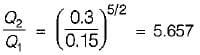QUESTION: 7

The discharge through a Cipolletti weir is given by where symbols have their, usual meanings.

Solution:Use Code STAYHOME200 and get INR 200 additional OFF Use Coupon Code